# Implement your own Riemannian Geometry#

Geomstats provides several Riemannian manifolds in its geometry folder. Yet, the manifold that you are interested in might not be available there.

This notebook shows how to use Riemannian geometry on any manifold defined by an immersion into a Euclidean space, such as high-dimensional surfaces immersed in a Euclidean space.

Specifically, we focus on the case of an embedded manifold $$M$$ that can be defined by a map f: $$f: M \rightarrow \mathbb{R}^n$$ called the immersion, whose differential $$df_x$$ is injective for all $$x \in M$$.

This immersion allows to define the pull-back metric $$g$$ on $$M$$, as:

$\begin{split}g : T_xM \times T_x M \rightarrow \mathbb{R}\\ u, v \rightarrow <df_x.u, df_x.v>\end{split}$

where $$<,>$$ represents the Euclidean inner-product of the embedding space.

The pull-back metric gives a structure of Riemannian manifold to $$M$$. In particular, we can compute the Riemannian exp and log maps, the Riemannian distance, the Riemannian parallel transport, etc.

This notion illustrates the computation of the pull-back metric, using the class PullbackMetric from geomstats, on two embedded manifolds: - the 2-sphere $$S^2$$ embedded in $$\mathbb{R}^3$$, - a surface defined by: $$x, y \rightarrow z = x^2 + y^2$$ embedded in $$\mathbb{R}^3$$.

## Setup#

 In :

import os
import sys
import time
import warnings

sys.path.append(os.path.dirname(os.getcwd()))
warnings.filterwarnings("ignore")

 In :

import matplotlib.pyplot as plt

import geomstats.visualization as viz

INFO: Using autograd backend

 In :

import geomstats.backend as gs


We import the main structure used in this notebook: the PullbackMetric:

 In :

from geomstats.geometry.pullback_metric import PullbackMetric


## Immersed manifolds: the example of the 2-sphere#

We first consider the simple example of the 2-sphere. We define the immersion of the 2-sphere $$S^2$$ into the Euclidean space $$\mathbb{R}^3$$ as follows:

$\begin{split}f : S^2 \rightarrow \mathbb{R}^3\\ (\theta, \phi) \rightarrow (\cos\phi.\sin\theta, \sin\phi.\sin\theta, \cos\theta)\end{split}$
 In :

def sphere_immersion(spherical_coords):
theta = spherical_coords[..., 0]
phi = spherical_coords[..., 1]
return gs.array(
[gs.cos(phi) * gs.sin(theta), gs.sin(phi) * gs.sin(theta), gs.cos(theta)]
)


For the purpose of visualizing the results in the embedding space $$\mathbb{R}^3$$, we will need the jacobian of the immersion, which we compute here:

 In :

jac_sphere_immersion = gs.autodiff.jacobian(sphere_immersion)


We use the PullbackMetric structure to define the Riemannian metric on $$S^2$$ from the immersion.

Note that the Riemannian metric on the sphere is already implemented in Geomstats using closed forms with the class Hypersphere. However, this notebook showcases how we can recover the computations of Riemanian geometry by only relying on the immersion.

 In :

sphere_metric = PullbackMetric(dim=2, embedding_dim=3, immersion=sphere_immersion)


Now, we can access the methods from any Riemannian metric, i.e. the Riemannian exp and log maps, the parallel transport, etc.

We first show the computation of the Riemannian exp map of a tangent vector at a point.

 In :

point_a = gs.array([gs.pi / 2.0, -gs.pi / 2.0])
tangent_vec = gs.array([0.0, gs.pi / 3.0])

end_point = sphere_metric.exp(tangent_vec=tangent_vec, base_point=point_a)
print(end_point)

[ 1.57079633 -0.52359878]


And visualize the result of the Riemannian exp map in the embedding space $$\mathbb{R}^3$$:

 In :

%matplotlib notebook

# We immerse the points and the tangent vector in R^3

immersed_point_a = sphere_immersion(point_a)
immersed_tangent_vec = gs.matvec(jac_sphere_immersion(point_a), tangent_vec)
immersed_end_point = sphere_immersion(end_point)

# We plot our results

fig = plt.figure(figsize=(8, 8))

viz.plot(immersed_point_a, ax=ax, space="S2", label="Initial point", s=80)

arrow = viz.Arrow3D(immersed_point_a, vector=immersed_tangent_vec)
arrow.draw(ax, color="black", label="Tangent vector")

viz.plot(immersed_end_point, ax=ax, space="S2", label="End point", s=80)

ax.set_title("Riemannian Exp map on the sphere")
ax.legend()
ax.grid(False)
plt.axis("off");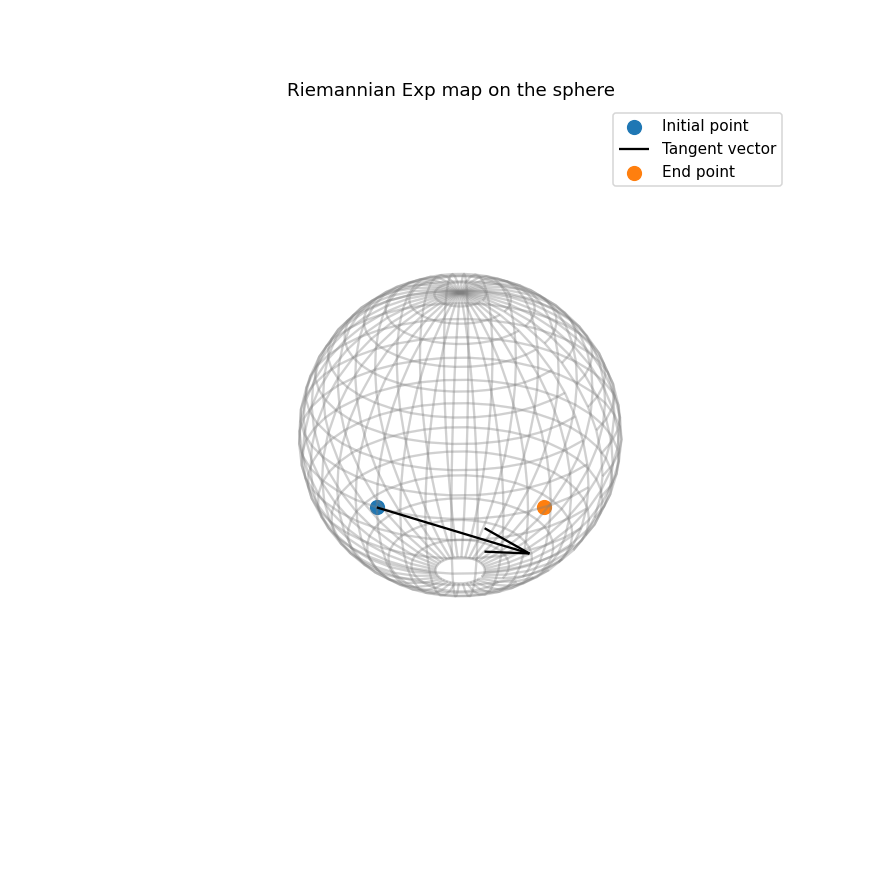Next, we show the computation of the parallel transport on the sphere. Note that step, n_steps, tol and alpha are integration parameters that control the efficiency-accuracy tradeoff of the computation.

Note: The accuracy of the computation varies for the different backends. We recommend using autograd, and we discourage the use of tensorflow.

 In :

point_a = gs.array([gs.pi / 2.0, -gs.pi / 2.0])
tangent_vec = gs.array([0.0, gs.pi / 3.0])
tangent_vec_to_transport = gs.array([gs.pi / 4.0, gs.pi / 3.0])

time_start = time.perf_counter()
tangent_vec=tangent_vec_to_transport,
direction=tangent_vec,
base_point=point_a,
step="euler",
n_steps=1,
tol=1e-6,
alpha=1,
)

time_elapsed = time.perf_counter() - time_start
print(f"Computing time for parallel transport: {time_elapsed:5.2f} secs")

display(parallel_transport)

transported_tangent_vec = parallel_transport["transported_tangent_vec"]
end_point = parallel_transport["end_point"]

Computing time for parallel transport:  4.71 secs

{'transported_tangent_vec': array([0.78539816, 1.04719755]),
'end_point': array([ 1.57079633, -0.52359878]),
'trajectory': [[]]}


We visualize the result of the parallel transport in the embedding space $$\mathbb{R}^3$$:

 In :

%matplotlib notebook

# We first immerse the points and tangent vectors into the embedding space R^3

immersed_point_a = sphere_immersion(point_a)
immersed_end_point = sphere_immersion(end_point)

immersed_tangent_vec = gs.matvec(jac_sphere_immersion(point_a), tangent_vec)
immersed_tangent_vec_to_transport = gs.matvec(
jac_sphere_immersion(point_a), tangent_vec_to_transport
)
immersed_transported_tangent_vec = gs.matvec(
jac_sphere_immersion(end_point), transported_tangent_vec
)

# We verify manually that the immersed tangent vector is actually tangent to the sphere
# as the plot can be sometimes misleading. We use the method of the Hypersphere class.

from geomstats.geometry.hypersphere import Hypersphere

sphere = Hypersphere(dim=2)
is_tangent = sphere.is_tangent(
immersed_transported_tangent_vec, base_point=immersed_end_point
)
print("is_tangent = ", is_tangent)

# We plot the results

fig = plt.figure(figsize=(8, 8))

viz.plot(immersed_point_a, ax=ax, space="S2", label="Initial point", s=80)

arrow = viz.Arrow3D(immersed_point_a, vector=immersed_tangent_vec)
arrow.draw(ax, color="black", label="Tangent vector")

arrow = viz.Arrow3D(immersed_point_a, vector=immersed_tangent_vec_to_transport)
arrow.draw(ax, color="red", label="Tangent vector to transport")

viz.plot(immersed_end_point, ax=ax, space="S2", label="End point", s=80)

arrow = viz.Arrow3D(immersed_end_point, vector=immersed_transported_tangent_vec)
arrow.draw(ax, color="orange", label="Transported tangent vector")

ax.set_title("Riemannian parallel transport on the sphere")
ax.legend()
ax.grid(False)
plt.axis("off");

is_tangent =  True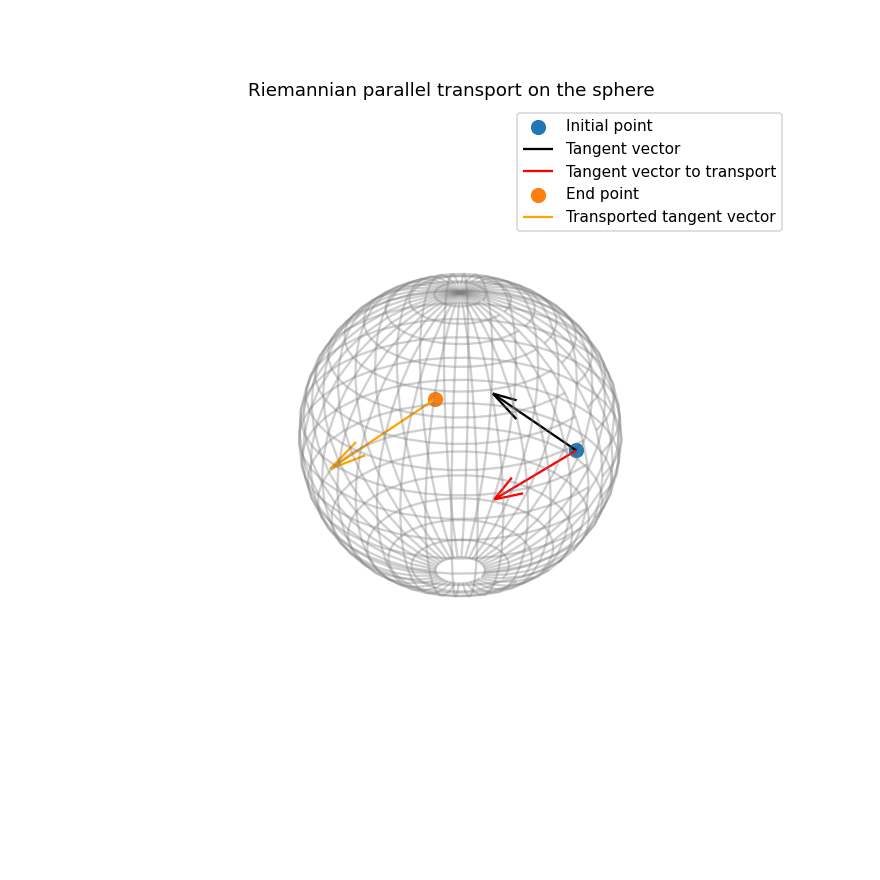## Immersed manifolds: the example of a surface defined by its graph#

We consider the example of a 2D surface immersed in $$\mathbb{R}^3$$. The surface is defined by its graph:

$x, y \rightarrow z = x^2 + y^2$

which leads to the following immersion into $$\mathbb{R}^3$$:

$\begin{split}f : S^2 \rightarrow \mathbb{R}^3\\ (x, y) \rightarrow (x, y, x^2 + y^2)\end{split}$

We first implement the graph and the immersion:

 In :

def surface_graph(x, y):
return x**2 + y**2

def surface_immersion(intrinsic_coords):
x = intrinsic_coords[..., 0]
y = intrinsic_coords[..., 1]
return gs.transpose(gs.array([x, y, surface_graph(x, y)]))


For the purpose of visualizing the results in the embedding space ℝ3 , we will need the jacobian of the immersion, which we compute here:

 In :

jac_surface_immersion = gs.autodiff.jacobian(surface_immersion)


We also add a utility function to visualization the surface in 3D:

 In :

%matplotlib notebook

def plot_surface(alpha=1.0, ax=None):
if ax is None:
fig = plt.figure()

x = y = gs.arange(-3.0, 3.0, 0.1)
X, Y = gs.meshgrid(x, y)
zs = gs.array(surface_graph(gs.flatten(X), gs.flatten(Y)))
Z = gs.reshape(zs, X.shape)

ax.plot_surface(gs.to_numpy(X), gs.to_numpy(Y), gs.to_numpy(Z), alpha=alpha)

ax.set_xlabel("X")
ax.set_ylabel("Y")
ax.set_zlabel("Zm")
plt.show()
return ax

ax = plot_surface()
ax.grid(False)
plt.axis("off");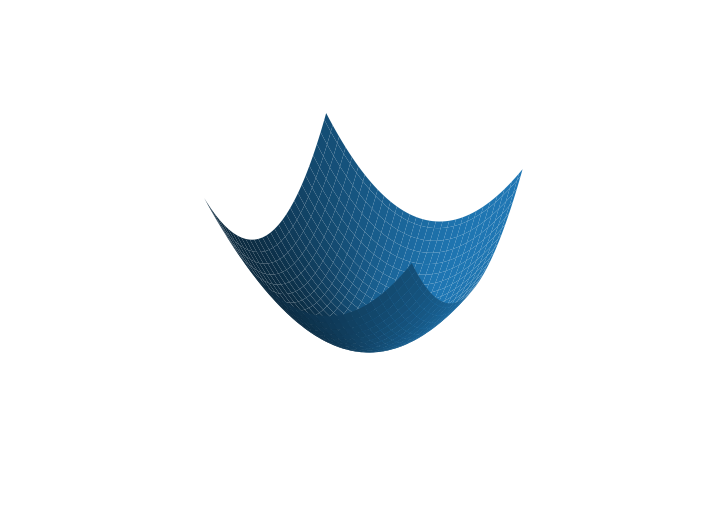We use the PullbackMetric structure to define the Riemannian metric on the surface from the immersion.

 In :

surface_metric = PullbackMetric(dim=2, embedding_dim=3, immersion=surface_immersion)


Now, we can access the methods from any Riemannian metric, i.e. the Riemannian exp and log maps, the parallel transport, etc.

We show the computation of the Riemannian exp map:

 In :

point_a = gs.array([-2.0, -2.0])
tangent_vec = gs.array([0.0, 1.0])

end_point = surface_metric.exp(tangent_vec=tangent_vec, base_point=point_a)
print(end_point)

[-1.85193664 -0.8761551 ]


And visualize the result:

 In :

%matplotlib notebook

# We first immerse the points and tangent vector into the embedding space R^3

immersed_point_a = surface_immersion(point_a)
immersed_tangent_vec = gs.matvec(jac_surface_immersion(point_a), tangent_vec)
immersed_end_point = surface_immersion(end_point)

two_points = gs.vstack([immersed_point_a, immersed_end_point])

# We plot the results

ax = plot_surface(alpha=0.3)

ax.plot(
immersed_point_a,
immersed_point_a,
immersed_point_a,
label="Initial point",
marker="o",
linestyle="None",
)

arrow = viz.Arrow3D(immersed_point_a, vector=immersed_tangent_vec)
arrow.draw(ax, color="black", label="Tangent vector")

ax.plot(
immersed_end_point,
immersed_end_point,
immersed_end_point,
label="End point",
marker="o",
linestyle="None",
)

ax.set_title("Riemannian exponential map on a surface")
ax.legend()
ax.grid(False)
plt.axis("off");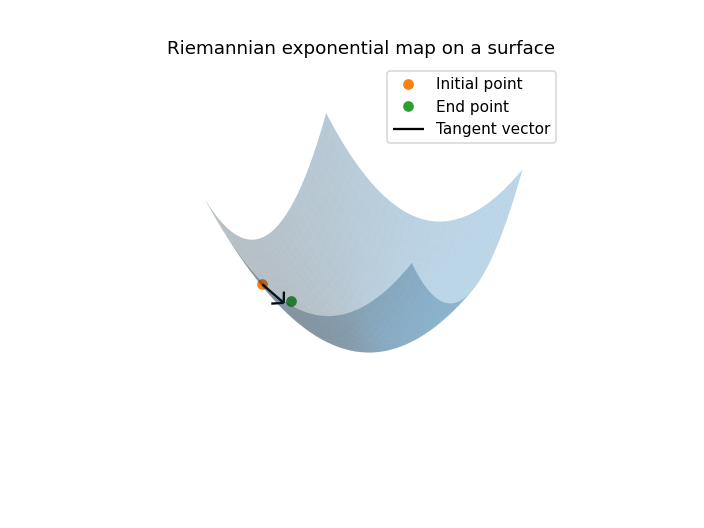Next, we show the computation of the parallel transport on the surface. Again, note that step, n_steps, tol and alpha are integration parameters that control the efficiency-accuracy tradeoff of the computation.

 In :

point_a = gs.array([-2.0, -2.0])
tangent_vec = gs.array([0.0, 1.0])
tangent_vec_to_transport = gs.array([-0.6, 0.6])

time_start = time.perf_counter()
tangent_vec=tangent_vec_to_transport,
direction=tangent_vec,
base_point=point_a,
step="rk4",
n_steps=1,
tol=1e-14,
alpha=2,
)
time_elapsed = time.perf_counter() - time_start
print(f"Computing time for parallel transport: {time_elapsed:5.2f} secs")

display(parallel_transport)

transported_tangent_vec = parallel_transport["transported_tangent_vec"]
end_point = parallel_transport["end_point"]

Computing time for parallel transport:  4.40 secs

{'transported_tangent_vec': array([-0.40316376,  0.73456928]),
'end_point': array([-1.85193664, -0.8761551 ]),
'trajectory': [[]]}


We visualize the result of the parallel transport.

 In :

%matplotlib notebook

# We first immerse the points and tangent vectors into the embedding space R^3

immersed_point_a = surface_immersion(point_a)
immersed_tangent_vec = gs.matvec(jac_surface_immersion(point_a), tangent_vec)
immersed_tangent_vec_to_transport = gs.matvec(
jac_surface_immersion(point_a), tangent_vec_to_transport
)
immersed_end_point = surface_immersion(end_point)
immersed_transported_tangent_vec = gs.matvec(
jac_surface_immersion(end_point), transported_tangent_vec
)

# We plot the results

ax = plot_surface(alpha=0.3)

ax.plot(
immersed_point_a,
immersed_point_a,
immersed_point_a,
label="Initial point",
marker="o",
color="orange",
)

arrow = viz.Arrow3D(immersed_point_a, vector=immersed_tangent_vec_to_transport)
arrow.draw(ax, color="orange", label="Tangent vector to transport")

arrow = viz.Arrow3D(immersed_point_a, vector=immersed_tangent_vec)
arrow.draw(ax, color="black", label="Tangent vector")

ax.plot(
immersed_end_point,
immersed_end_point,
immersed_end_point,
label="End point",
marker="o",
color="green",
)

arrow = viz.Arrow3D(immersed_end_point, vector=immersed_transported_tangent_vec)
arrow.draw(ax, color="green", label="Transported tangent vector")

ax.set_title("Riemannian parallel transport on a surface")
ax.legend()
ax.grid(False)
plt.axis("off");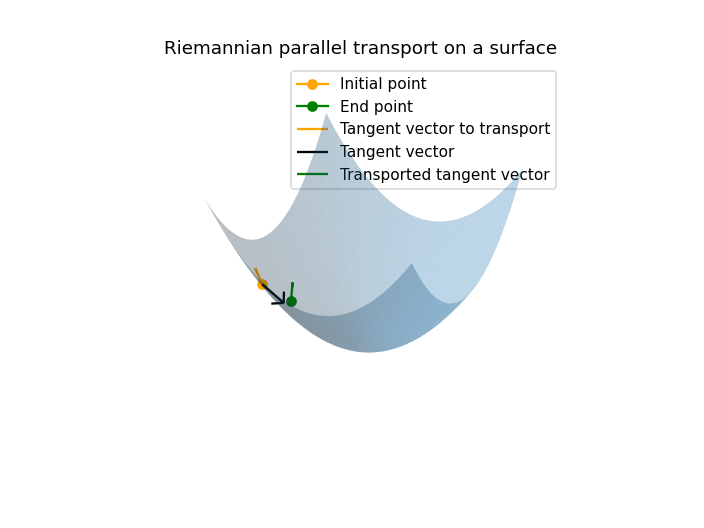In [ ]: